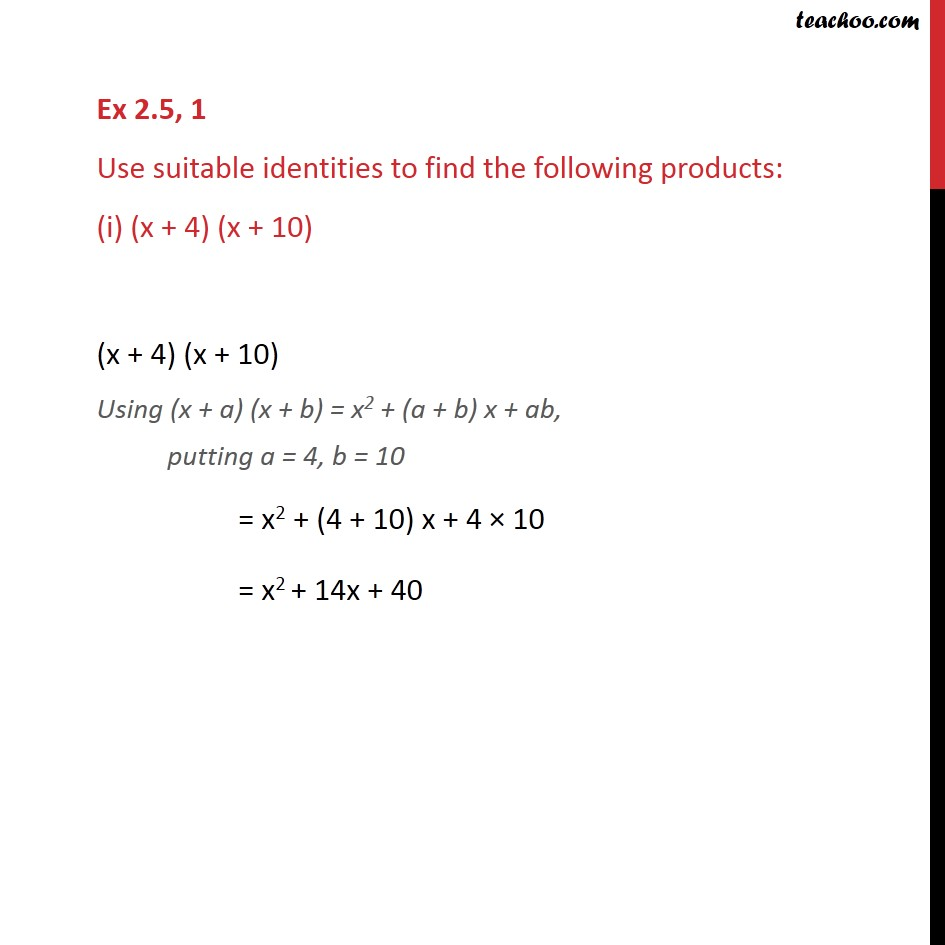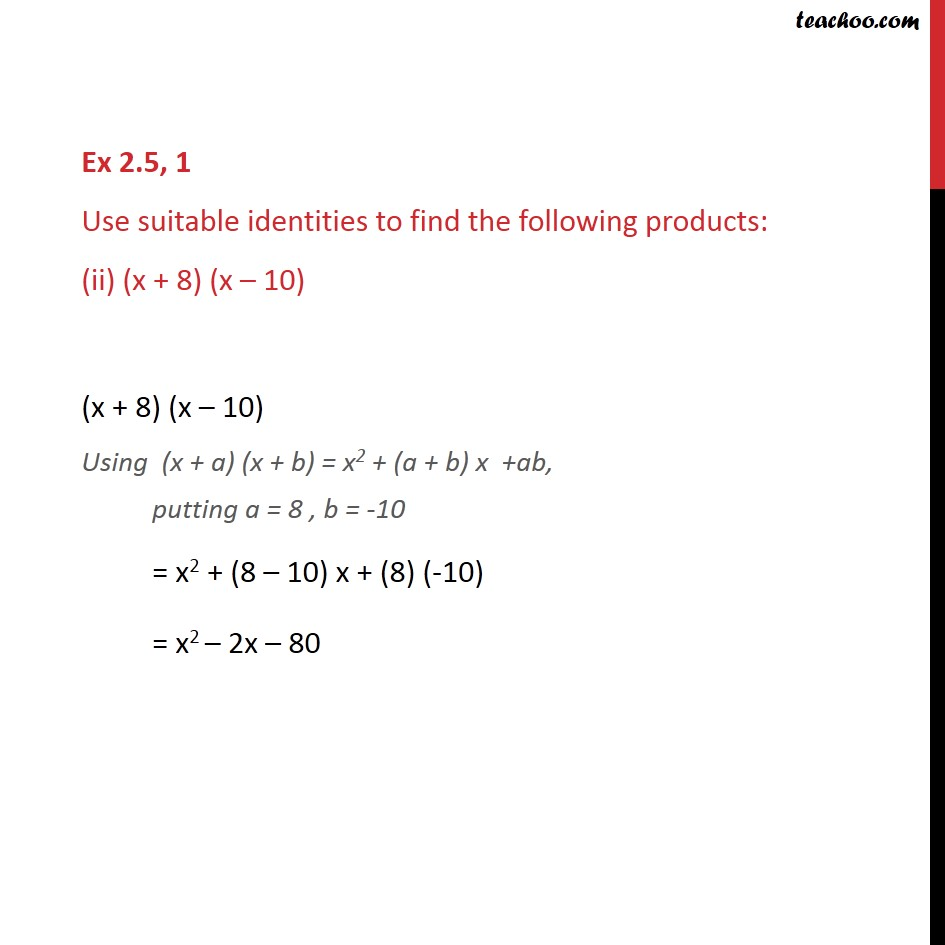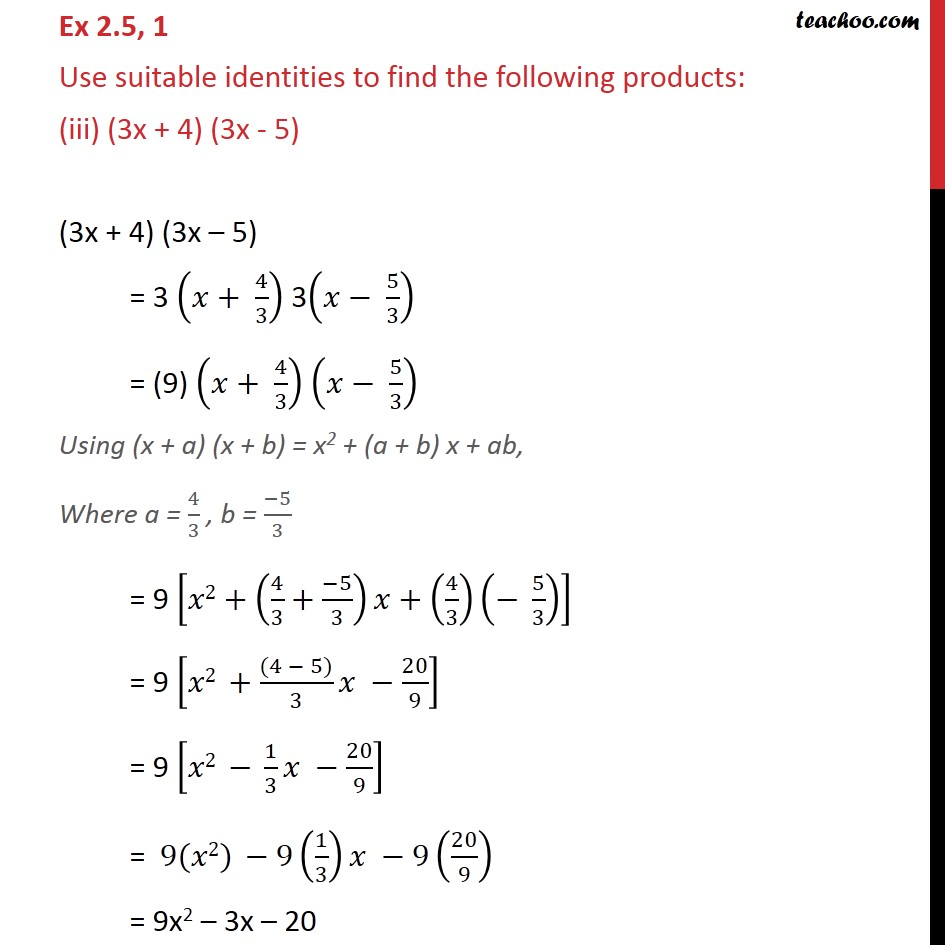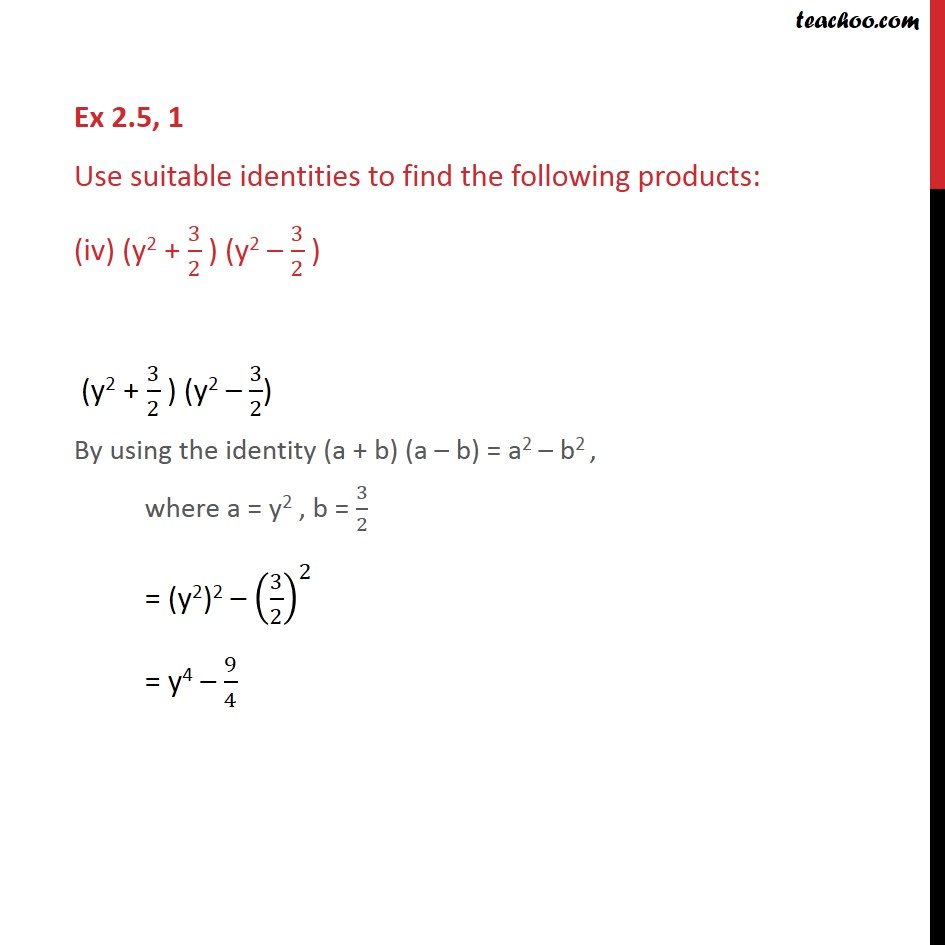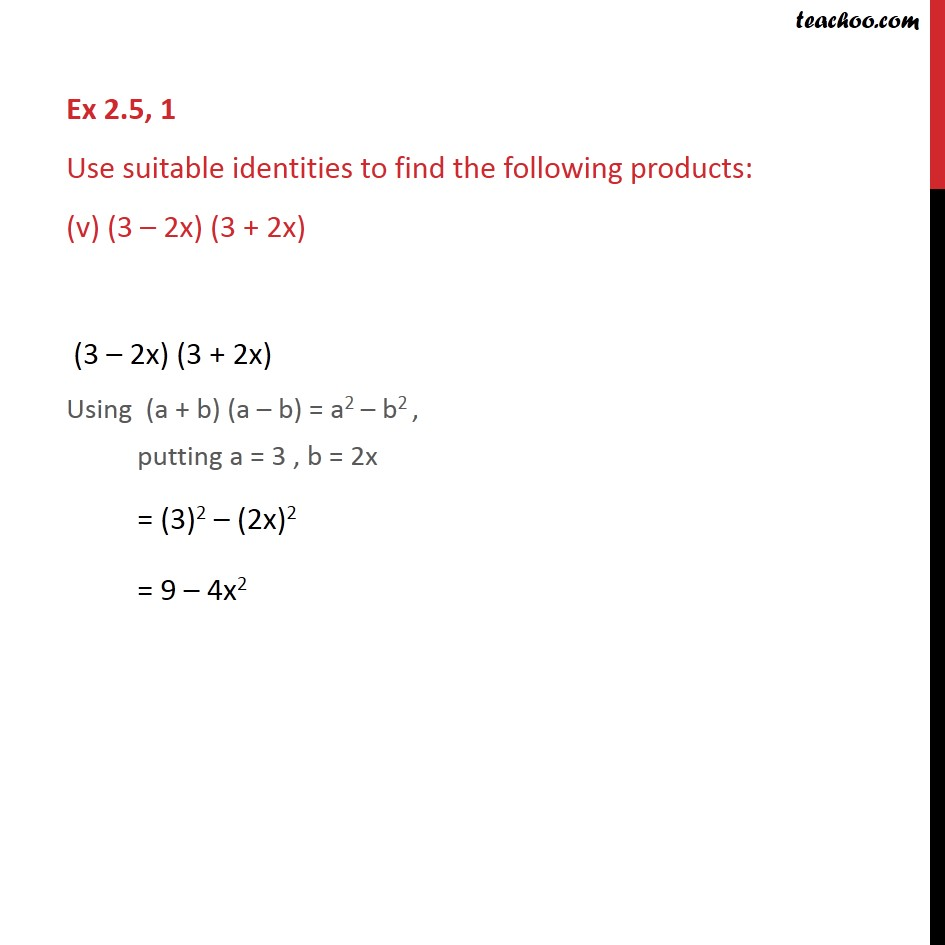1. Chapter 2 Class 9 Polynomials
2. Serial order wise
3. Ex 2.5

Transcript

Ex 2.5, 1 Use suitable identities to find the following products: (i) (x + 4) (x + 10) (x + 4) (x + 10) Using (x + a) (x + b) = x2 + (a + b) x + ab, putting a = 4, b = 10 = x2 + (4 + 10) x + 4 × 10 = x2 + 14x + 40 Ex 2.5, 1 Use suitable identities to find the following products: (ii) (x + 8) (x – 10) (x + 8) (x – 10) Using (x + a) (x + b) = x2 + (a + b) x +ab, putting a = 8 , b = -10 = x2 + (8 – 10) x + (8) (-10) = x2 – 2x – 80 Ex 2.5, 1 Use suitable identities to find the following products: (iii) (3x + 4) (3x - 5) (3x + 4) (3x – 5) = 3 (𝑥+ 4/3) 3(𝑥− 5/3) = (9) (𝑥+ 4/3) (𝑥− 5/3) Using (x + a) (x + b) = x2 + (a + b) x + ab, Where a = 4/3 , b = (−5)/3 = 9 [𝑥2+(4/3+(−5)/3)𝑥+(4/3)(− 5/3)] = 9 [𝑥2 +((4 − 5))/3 𝑥 −20/9] = 9 [𝑥2 − 1/3 𝑥 −20/9] = 9(𝑥2) −9(1/3)𝑥 −9(20/9) = 9x2 – 3x – 20 Ex 2.5, 1 Use suitable identities to find the following products: (iv) (y2 + 3/2 ) (y2 – 3/2 ) (y2 + 3/2 ) (y2 – 3/2) By using the identity (a + b) (a – b) = a2 – b2 , where a = y2 , b = 3/2 = (y2)2 – (3/2)^2 = y4 – 9/4 Ex 2.5, 1 Use suitable identities to find the following products: (v) (3 – 2x) (3 + 2x) (3 – 2x) (3 + 2x) Using (a + b) (a – b) = a2 – b2 , putting a = 3 , b = 2x = (3)2 – (2x)2 = 9 – 4x2

Ex 2.5

Chapter 2 Class 9 Polynomials
Serial order wise

About the AuthorDavneet Singh
Davneet Singh is a graduate from Indian Institute of Technology, Kanpur. He has been teaching from the past 10 years. He provides courses for Maths and Science at Teachoo.